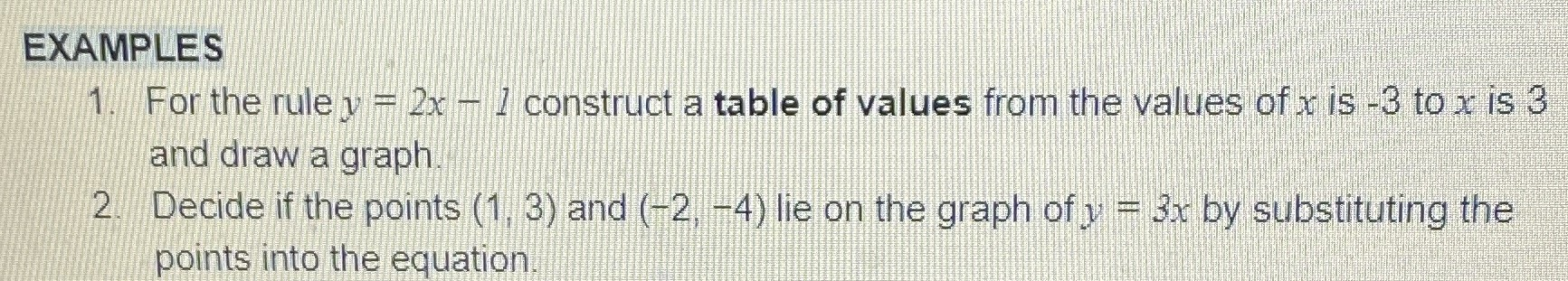### ¿Todavía tienes preguntas de matemáticas?

Pregunte a nuestros tutores expertos
Algebra
Pregunta1. For the rule $$y = 2 x - 1$$ construct a table of values from the values of $$x$$ is $$- 3$$ to $$x$$ is $$3$$ and draw a graph 2. Decide if the points $$( 1,3 )$$ and $$( - 2 , - 4 )$$ lie on the graph of $$y = 3 x$$ by substituting the points into the equation.